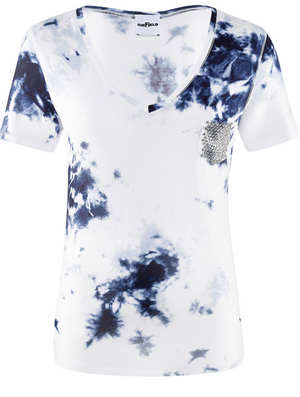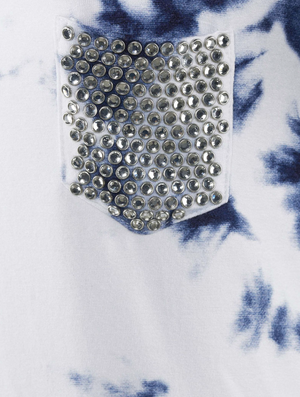Airfield

# Tie Dye Pocket Tee

Regular price \$245 now \$171.50 Unit price  per

Shipping calculated at checkout.
• 97% viscose 3% elastane
• Machine wash cold
• Hang to dry
• Short sleeve
• Sequin pocket on left side
• Fits true to size

Size Guide

• 34 = 4 = XS
• 36 = 6 = S
• 38 = 8 = M
• 40 = 10 = M
• 42 = 12 = L
• 44 = 14 = L
• 46 = 16 = XL